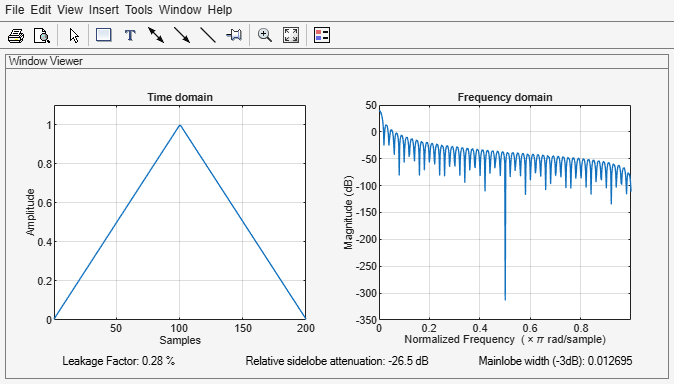# triang

Triangular window

## Syntax

``w = triang(L)``

## Description

example

````w = triang(L)` returns an `L`-point triangular window.```

## Examples

collapse all

Create a 200-point triangular window. Display the result using `wvtool`.

```L = 200; w = triang(L); wvtool(w)```## Input Arguments

collapse all

Window length, specified as a positive integer.

Data Types: `single` | `double`

## Output Arguments

collapse all

Triangular window, returned as a column vector.

## Algorithms

The coefficients of a triangular window are:

For L odd:

`$w\left(n\right)=\left\{\begin{array}{ll}\frac{2n}{L+1}\hfill & 1\le n\le \frac{L+1}{2}\hfill \\ 2-\frac{2n}{L+1}\hfill & \frac{L+1}{2}+1\le n\le L\hfill \end{array}$`

For L even:

`$w\left(n\right)=\left\{\begin{array}{ll}\frac{\left(2n-1\right)}{L}\hfill & 1\le n\le \frac{L}{2}\hfill \\ 2-\frac{\left(2n-1\right)}{L}\hfill & \frac{L}{2}+1\le n\le L\hfill \end{array}$`

 Oppenheim, Alan V., Ronald W. Schafer, and John R. Buck. Discrete-Time Signal Processing. Upper Saddle River, NJ: Prentice Hall, 1999.